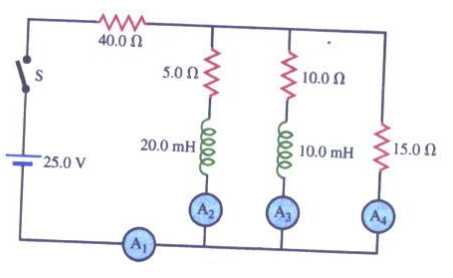# Problem: In the circuit shown in the figure below, switch S is closed at a time t = 0.Find the reading of each ammeter after a long period of time has passed with the switch closed.

###### FREE Expert Solution

Ohm's law:

$\overline{){\mathbf{V}}{\mathbf{=}}{\mathbf{i}}{\mathbf{R}}}$

Equivalent resistance for resistors in series:

$\overline{){{\mathbf{R}}}_{{\mathbf{eq}}}{\mathbf{=}}{{\mathbf{R}}}_{{\mathbf{1}}}{\mathbf{+}}{{\mathbf{R}}}_{{\mathbf{2}}}{\mathbf{+}}{\mathbf{.}}{\mathbf{.}}{\mathbf{.}}{\mathbf{+}}{{\mathbf{R}}}_{{\mathbf{n}}}}$

Equivalent resistance for resistors in parallel:

$\overline{)\frac{\mathbf{1}}{{\mathbf{R}}_{\mathbf{eq}}}{\mathbf{=}}\frac{\mathbf{1}}{{\mathbf{R}}_{\mathbf{1}}}{\mathbf{+}}\frac{\mathbf{1}}{{\mathbf{R}}_{\mathbf{2}}}{\mathbf{+}}{\mathbf{.}}{\mathbf{.}}{\mathbf{.}}{\mathbf{+}}\frac{\mathbf{1}}{{\mathbf{R}}_{\mathbf{n}}}}$

After a long period of time, an inductor acts as a short circuit.

The 5.0Ω, the 10.0Ω, and the 15.0Ω resistor are in parallel.

79% (295 ratings)###### Problem DetailsIn the circuit shown in the figure below, switch S is closed at a time t = 0.

Find the reading of each ammeter after a long period of time has passed with the switch closed.

Frequently Asked Questions

What scientific concept do you need to know in order to solve this problem?

Our tutors have indicated that to solve this problem you will need to apply the LR Circuits concept. You can view video lessons to learn LR Circuits. Or if you need more LR Circuits practice, you can also practice LR Circuits practice problems.